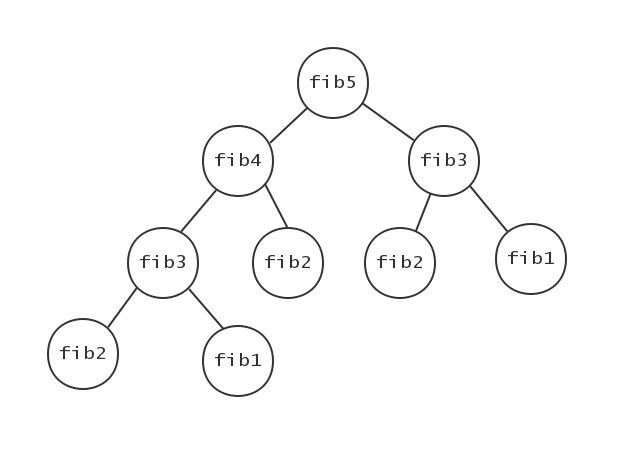•MoshiCoCo

# dynamic-programming note (动态规划专题)

## 开始DP之前

$${1,1,2,3,5,8,13,21····}$$

$$fib(n) =\left. \begin \end1 & n=1,2\fib(n-1)+fib(n-2) & n>2\ \right.$$## 关于0/1 Knapsack Problem 的思考过程

### 样例输入

3 5
2 3
3 5
4 7


### 样例输出

8


### 数据规模和约定

$1<=N<=200,M<=5000.$

## 首先是状态转移方程

$N$物品的件数
$Ni$第 $i$ 件物品
$V$背包的总容量
$v$当前背包的容量
$Ci$第 $i$ 个物品消耗的容量
$Wi$第 $i$ 个物品获取的价值

$$Choice =\left. \begin \endCi>v & 不偷 \Ci<v & 偷或者不偷 \ \right.$$

$$Max =\left. \begin F[i,v]=F[i-1,v]&不偷\ F[i,v]=F[i-1,v-Ci] \end+Wi&偷\ \right.$$

$$F[i,v]=F[i-1,v]$$

$$F[i,v]=F[i-1,v-Ci]+Wi$$

$$Max =\left. \begin F[i,v]=F[i-1,v]&装不下，不偷\ F[i,v]=F[i-1,v]&装的下，不偷\ F[i,v]=F[i-1,v-Ci] \end+Wi&装的下，偷\ \right.$$

## 算法设计和实现

### 空间复杂度$O(NV)$

F[N+1][V+1]={0};初始化数组都为0
for i←1 to N //从1号物品到N号物品
for v←Ci to V //背包空间从1-V
if(Ci>v) //第i个物品的重量大于当前背包容量
F[i,v]=F[i-1,v]; //装不下，不拿
else //可以拿的时候,取拿或者不拿的最优解。
F[i,v]=max(F[i-1,v],F[i-1,v-Ci]+Wi);


$v→V$01234567891011121314151617181920
$Ni$$Ci$$Wi$000000000000000000000
125005555555555555555555
2370057712121212121212121212121212121212
3640057712121212121216161616161616161616
4380058813151520202020202024242424242424
5450058813151520202020252525252525292929

### 空间复杂度$O(V)$

$$Max=\left. \begin dp[v]=dp[v]&装不下，不偷\ dp[v]=dp[v]&装的下，不偷\ dp[v]=dp[v-Ci] \end+Wi&装的下，偷\ \right.$$

dp[V+1]={0}; //初始化数组都为0
for i←1 to N //从1号物品到N号物品
for v←V to Ci && v>=Ci //背包空间从V到1,v大于等于Ci，反之不可拿，
//这里优化掉了，因为不拿的时候值不用更新，所以只考虑拿的时候值才会更新
dp[v]=max(dp[v],dp[v-C[i]]+W[i]); //可以拿时取最优


## 实现代码

#include <iostream>
#define MAXN 201
#define MAXM 5001
using namespace std;

int F[MAXN][MAXM]= {{0}};

int main () {
int N,V;
cin>>N>>V;
int dp[V+1]= {0};
int C[N+1]= {0},W[V+1]= {0};//花费C（单个物体的重量），获得价值W
//数据读入
for(int i=1; i<=N; i++) {
cin>>C[i]>>W[i];
}

//空间复杂度O(NV)
int i,v;//第i件物品，当前背包容量v
for(i=1; i<=N; i++) {//第1到第N件物品
for(v=C[i]; v<=V; v++) {//背包空间C[i]到V;
if(C[i]>v) {//能不能放下
F[i][v]=F[i-1][v];//不放
} else {
F[i][v]=max(F[i-1][v],F[i-1][v-C[i]]+W[i]);//取放和不放的最优解
}
}
}
std::cout<<F[N][V]<<endl;
//空间复杂度O(V)
for(i=1; i<=N; i++) {
for(v=V; v>=C[i]; v--) {
dp[v]=max(dp[v],dp[v-C[i]]+W[i]);
}
}
std::cout<<dp[V]<<endl;
//输出二维数组F
cout<<"O(NV)"<<endl;

for(int i=1; i<=N; i++) {
for(int j=1; j<=V; j++) {
cout<<F[i][j]<<" ";
}
cout<<endl;
}
//输出一维数组dp
cout<<"O(N)"<<endl;
for(int i=1; i<=V; i++) {
cout<<dp[i]<<" ";
}
cout<<endl;
return 0;
}